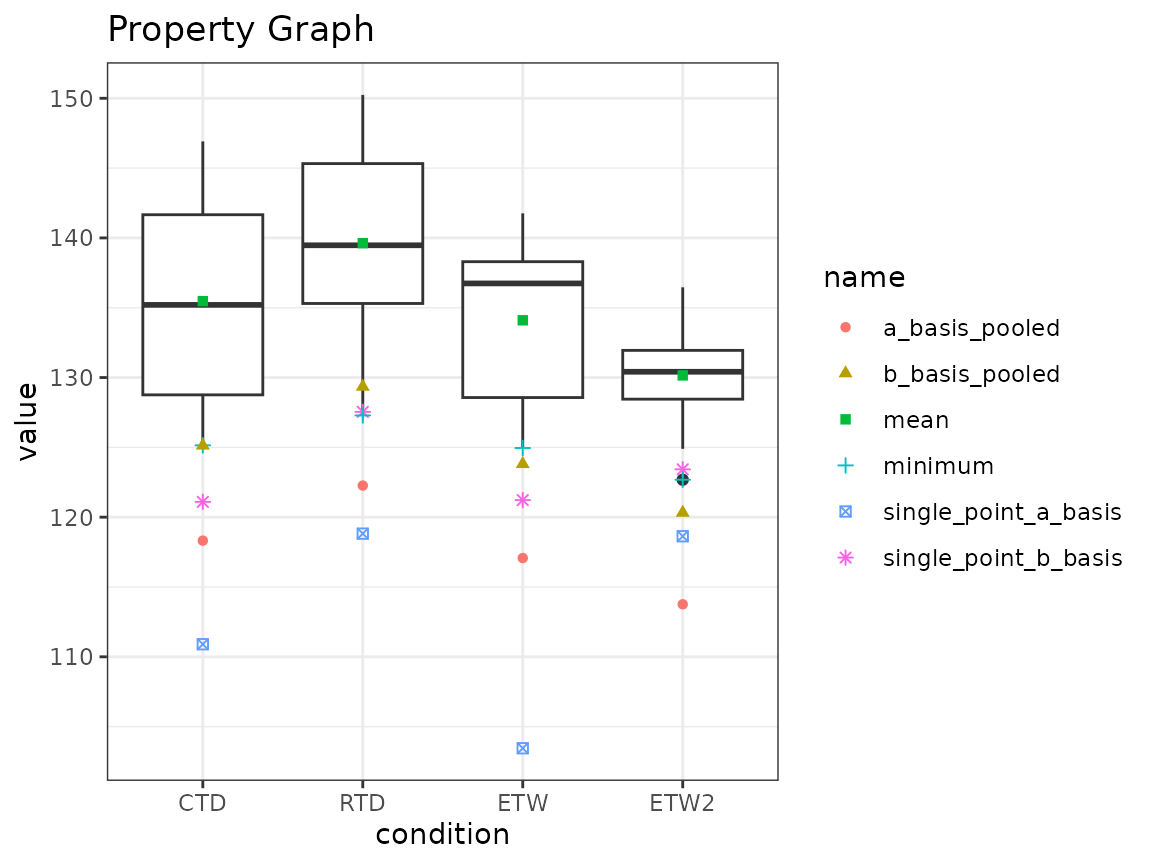This vignette demonstrates how to create some of the graphs commonly used when analyzing composite material data. Here, we rely on the ggplot2 package for graphing. This package can be loaded either on its own, or through the tidyverse meta-package, which also includes packages such as dplyr that we will also use.

We’ll need to load a few packages in order to proceed.

library(dplyr)
library(ggplot2)
library(tidyr)
library(cmstatr)

Throughout this vignette, we’ll use one of the example data sets that comes with cmstatr and we’ll focus on the warp-tension data as an example. We’ll load the example data in a variable as follows. By default the condition will be in an arbitrary order, but throughout the visualization, we’ll want the conditions shown in a particular order (from coldest and driest to hottest and wettest). We can define the order of the conditions using the ordered function. For brevity, only the first few rows of the data set are displayed below.

dat <- carbon.fabric.2 %>%
filter(test == "WT") %>%
mutate(condition = ordered(condition, c("CTD", "RTD", "ETW", "ETW2")))

dat %>%
#>    test condition batch thickness nplies strength modulus failure_mode
#> 1    WT       CTD     A     0.112     14  142.817   9.285          LAT
#> 2    WT       CTD     A     0.113     14  135.901   9.133          LAT
#> 3    WT       CTD     A     0.113     14  132.511   9.253          LAT
#> 4    WT       CTD     A     0.112     14  135.586   9.150          LAB
#> 5    WT       CTD     A     0.113     14  125.145   9.270          LAB
#> 6    WT       CTD     A     0.113     14  135.203   9.189          LGM
#> 7    WT       CTD     A     0.113     14  128.547   9.088          LAB
#> 8    WT       CTD     B     0.113     14  127.709   9.199          LGM
#> 9    WT       CTD     B     0.113     14  127.074   9.058          LGM
#> 10   WT       CTD     B     0.114     14  126.879   9.306          LGM

We’ll then calculate the B-Basis value using the pooling by standard deviation method. This data set happens to fail some of the diagnostic tests, but for the purpose of this example, we’ll ignore those failures using the override argument.

b_basis_pooled <- dat %>%
basis_pooled_cv(strength, condition, batch,
override = c("between_group_variability",
"normalized_variance_equal"))

b_basis_pooled
#>
#> Call:
#> basis_pooled_cv(data = ., x = strength, groups = condition, batch = batch,
#>     override = c("between_group_variability", "normalized_variance_equal"))
#>
#> Distribution:  Normal - Pooled CV    ( n = 86, r = 4 )
#> The following diagnostic tests were overridden:
#>     between_group_variability,
#>     normalized_variance_equal
#> B-Basis:   ( p = 0.9 , conf = 0.95 )
#> CTD   125.1325
#> RTD   129.3447
#> ETW   123.809
#> ETW2  120.3191

The object returned from basis_pooled_cv contains a number of values. One value is a data.frame containing the groups (i.e. conditions) and the corresponding basis values. This looks like the following. We’ll use this in the visualizations.

b_basis_pooled$basis #> group value #> CTD CTD 125.1325 #> RTD RTD 129.3447 #> ETW ETW 123.8090 #> ETW2 ETW2 120.3191 # Batch Plots Batch plots are used to identify differences between batches. Simple batch plots can be created using box plots and adding horizontal lines for the basis values as follows. Note that the heavy line in the box of the box plot is the median, not the mean. The two hinges correspond with the first and third quantiles and the whiskers extend to the most extreme data point, or 1.5 times the inner quantile range. In the code below, we use the function rename to rename the column group to condition. The data.frame produced by basis_pooled_cv uses the columns value and group, but to match the data, we need the column with the conditions to be named condition. dat %>% ggplot(aes(x = batch, y = strength)) + geom_boxplot() + geom_jitter(width = 0.25) + geom_hline(aes(yintercept = value), data = b_basis_pooled$basis %>% rename(condition = group),
color = "blue") +
facet_grid(. ~ condition) +
theme_bw() +
ggtitle("Batch Plot")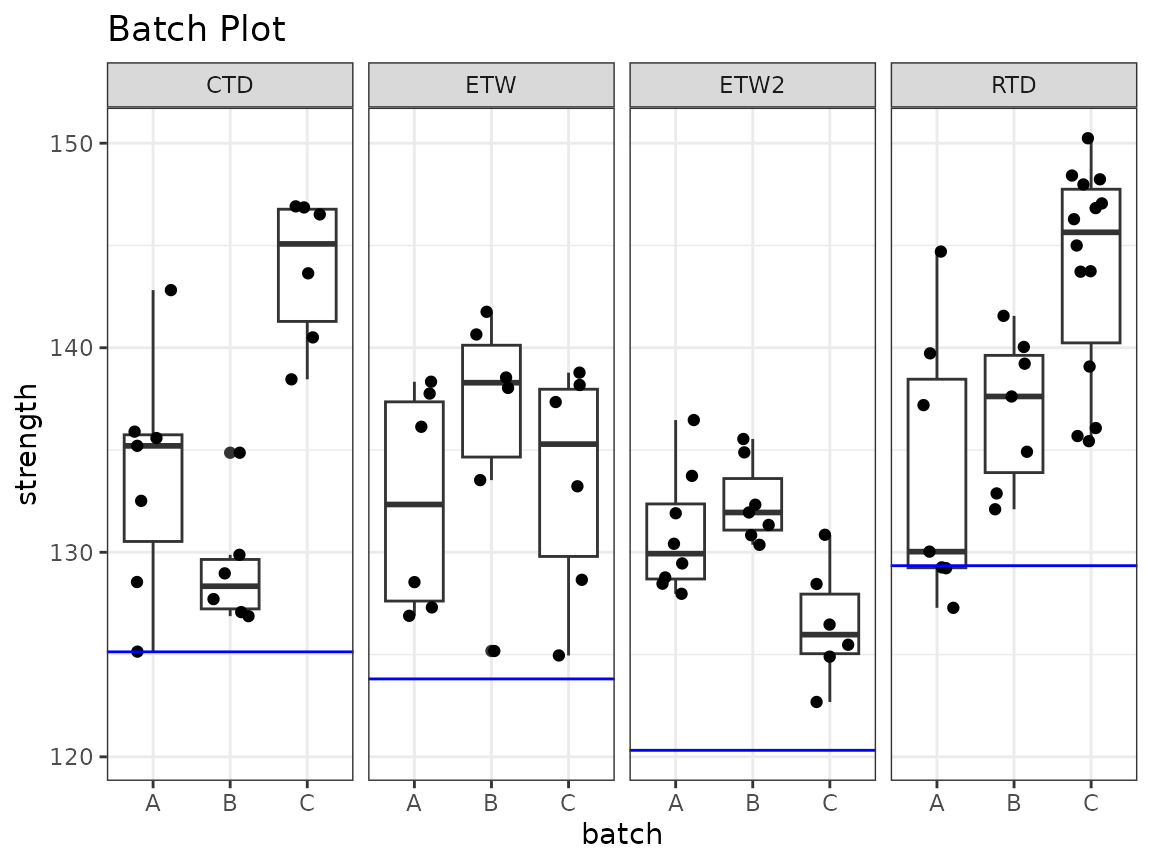# Quantile Plots

A quantile plot provides a graphical summary of sample values. This plot displays the sample values and the corresponding quantile. A quantile plot can be used to examine the symmetry and tail sizes of the underlying distribution. Sharp rises may indicate the presence of outliers.

dat %>%
ggplot(aes(x = strength, color = condition)) +
stat_ecdf(geom = "point") +
coord_flip() +
theme_bw() +
ggtitle("Quantile Plot")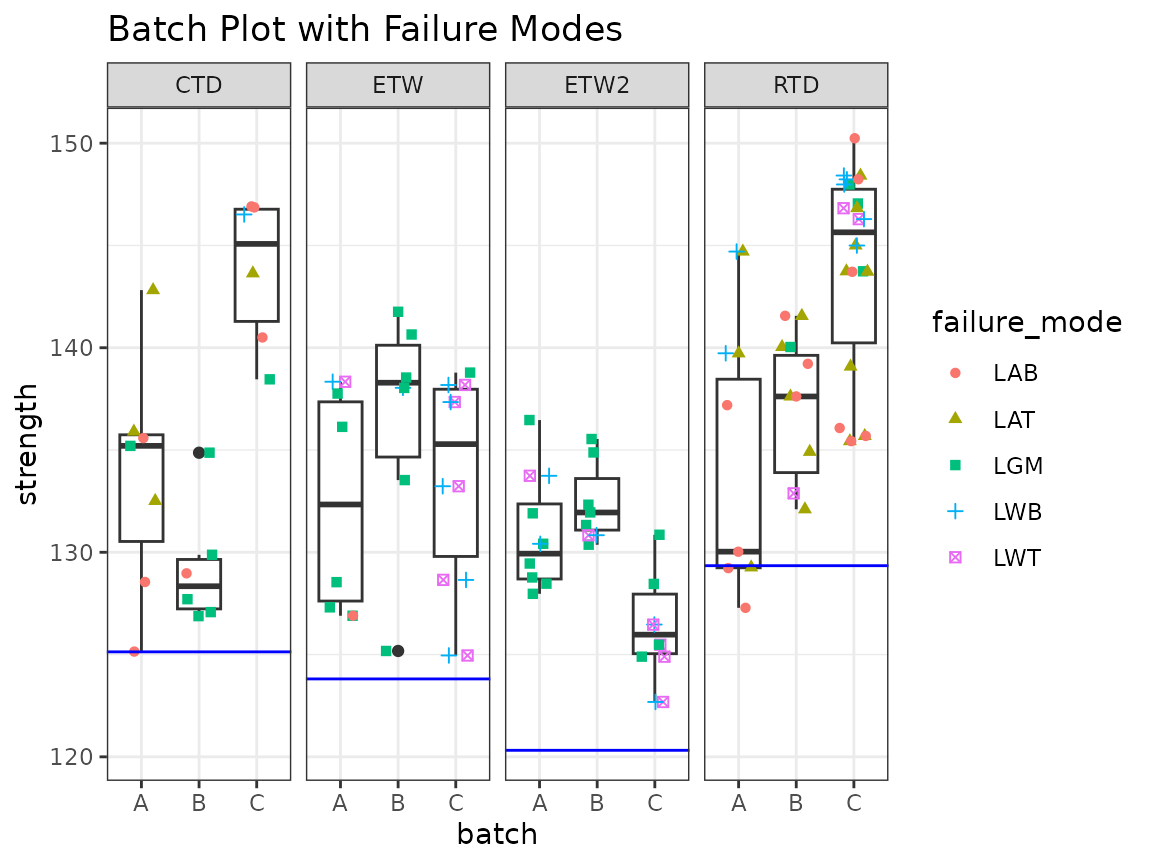# Normal Survival Function Plots

An empirical survival function, and the corresponding normal survival function can be plotted using two ggplot “stat” functions provided by cmstatr. In the example below, the empirical survival function is plotted for each condition, and the survival function for a normal distribution with the mean and variance from the data is also plotted (the survival function is 1 minus the cumulative distribution function). This type of plot can be used to identify how closely the data follows a normal distribution, and also to compare the distributions of the various conditions.

dat %>%
ggplot(aes(x = strength, color = condition)) +
stat_normal_surv_func() +
stat_esf() +
theme_bw() +
ggtitle("Normal Survival Function Plot")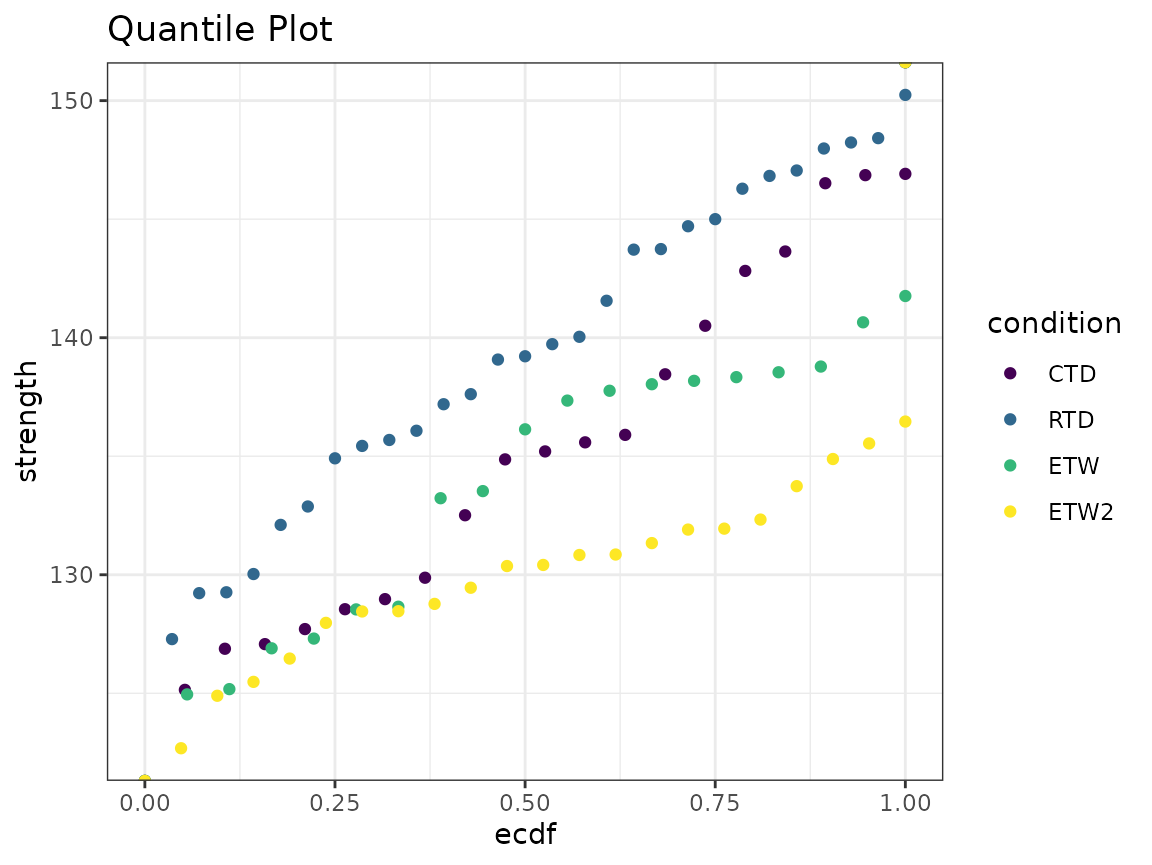# Normal Score Plots

The normal scores plot calculates the normal score and plots it against the normal score. Normal plots are useful to investigate distributions of the data.

dat %>%
group_by(condition) %>%
mutate(norm.score = scale(strength)) %>%
ggplot(aes(x = norm.score, y = strength, colour = condition)) +
geom_point() +
ggtitle("Normal Scores Plot") +
theme_bw()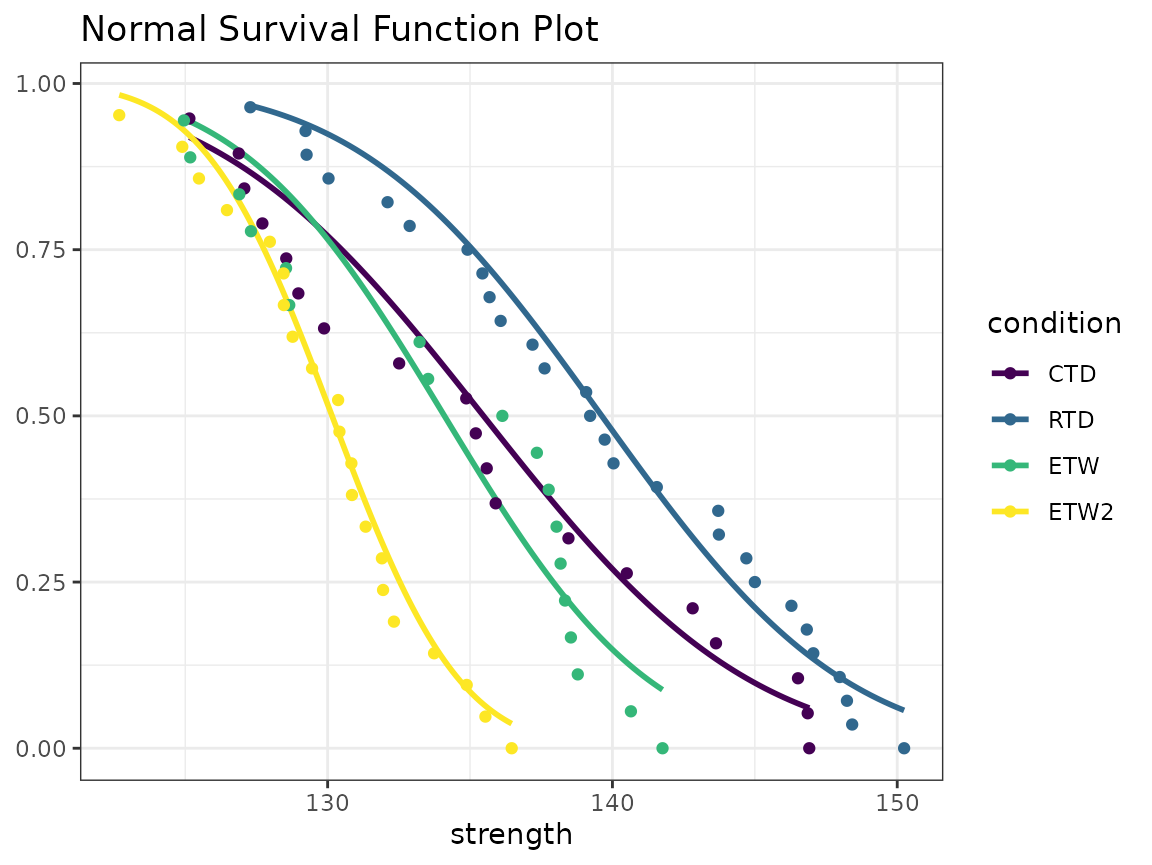# Q-Q Plots

A Q-Q plot compares the data against the theoretical quantiles for a particular distribution. A line is also plotted showing the normal distribution with mean and variance from the data. If the data exactly followed a normal distribution, all points would fall on this line.

dat %>%
ggplot(aes(sample = strength, colour = condition)) +
geom_qq() +
geom_qq_line() +
ggtitle("Q-Q Plot") +
theme_bw()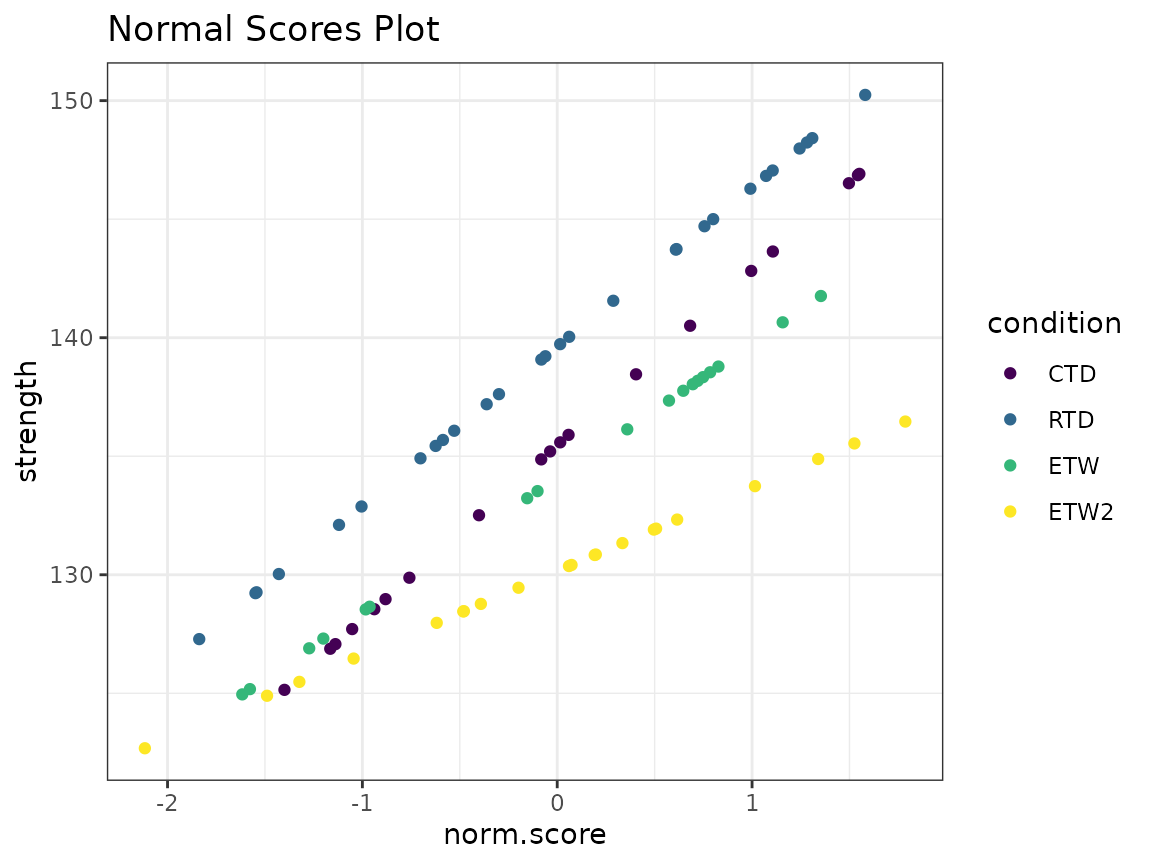# Property Plots

Property plots allow for a variety of properties for a group to be compared to other properties within the same group, as well as to other group properties. The properties included in this plot are A-Basis, B-Basis, Pooled A- and B-Basis, Pooled Modified CV (Coefficient of Variation) A- and B-Basis, Mean, and Min for each group.

The property plots will take a bit of work to construct.

First, the distribution of each group must be determined. Once the distribution has been determined, the proper basis calculation based on that distribution should be filled in below. We also have a column in the tables below for extra arguments to pass to the basis function, such as overrides required or the method for the basis_hk_ext function to use.

b_basis_fcn <- tribble(
~condition, ~fcn, ~args,
"CTD", "basis_normal", list(override = c("between_batch_variability")),
"RTD", "basis_normal", list(override = c("between_batch_variability")),
"ETW", "basis_hk_ext", NULL,
"ETW2", "basis_normal", list(override = c("between_batch_variability"))
)

a_basis_fcn <- tribble(
~condition, ~fcn, ~args,
"CTD", "basis_normal", list(override = c("between_batch_variability")),
"RTD", "basis_normal", list(override = c("between_batch_variability")),
"ETW", "basis_hk_ext", list(method = "woodward-frawley"),
"ETW2", "basis_normal", list(override = c("between_batch_variability"))
)

We’ll write a function that takes the data and information about the distribution and computes the single-point basis value. We’ll use this function for both A- and B-Basis, so we’ll add a parameter for the probability (0.90 or 0.99).

single_point_fcn <- function(group_x, group_batch, cond, basis_fcn, p) {
fcn <- basis_fcn$fcn[basis_fcn$condition == cond]
extra_args <- basis_fcn$args[basis_fcn$condition == cond]

args <- c(
list(x = group_x, batch = group_batch, p = p),
unlist(extra_args))
basis <- do.call(fcn, args)
basis$basis } single_point_results <- dat %>% group_by(condition) %>% summarise(single_point_b_basis = single_point_fcn( strength, batch, condition, b_basis_fcn, 0.90), single_point_a_basis = single_point_fcn( strength, batch, condition, a_basis_fcn, 0.99), minimum = min(strength), mean = mean(strength)) %>% mutate(condition = ordered(condition, c("CTD", "RTD", "ETW", "ETW2"))) #> summarise() ungrouping output (override with .groups argument) single_point_results #> # A tibble: 4 x 5 #> condition single_point_b_basis single_point_a_basis minimum mean #> <ord> <dbl> <dbl> <dbl> <dbl> #> 1 CTD 121. 111. 125. 135. #> 2 RTD 128. 119. 127. 140. #> 3 ETW 121. 103. 125. 134. #> 4 ETW2 123. 119. 123. 130. In the above code, we also ensure that the condition column is still in the order we expect. We’ve already computed the B-Basis of the data using a pooling method. We’ll do the same for A-Basis: a_basis_pooled <- dat %>% basis_pooled_cv(strength, condition, batch, p = 0.99, override = c("between_group_variability", "normalized_variance_equal")) a_basis_pooled #> #> Call: #> basis_pooled_cv(data = ., x = strength, groups = condition, batch = batch, #> p = 0.99, override = c("between_group_variability", "normalized_variance_equal")) #> #> Distribution: Normal - Pooled CV ( n = 86, r = 4 ) #> The following diagnostic tests were overridden: #> between_group_variability, #> normalized_variance_equal #> A-Basis: ( p = 0.99 , conf = 0.95 ) #> CTD 118.3205 #> RTD 122.2636 #> ETW 117.074 #> ETW2 113.7601 As we saw before, the returned object has a property called basis, which is a data.frame for the pooling methods. a_basis_pooled$basis
#>      group    value
#> CTD    CTD 118.3205
#> RTD    RTD 122.2636
#> ETW    ETW 117.0740
#> ETW2  ETW2 113.7601

We can take this data.frame and change the column names to suit our needs.

a_basis_pooled$basis %>% rename(condition = group, b_basis_pooled = value) #> condition b_basis_pooled #> CTD CTD 118.3205 #> RTD RTD 122.2636 #> ETW ETW 117.0740 #> ETW2 ETW2 113.7601 We can combine all these steps into one statement. We’ll also ensure that the conditions are listed in the order we want. a_basis_pooled_results <- a_basis_pooled$basis %>%
rename(condition = group,
a_basis_pooled = value) %>%
mutate(condition = ordered(condition, c("CTD", "RTD", "ETW", "ETW2")))

a_basis_pooled_results
#>   condition a_basis_pooled
#> 1       CTD       118.3205
#> 2       RTD       122.2636
#> 3       ETW       117.0740
#> 4      ETW2       113.7601

And the same thing for B-Basis:

b_basis_pooled_results <- b_basis_pooled\$basis %>%
rename(condition = group,
b_basis_pooled = value) %>%
mutate(condition = ordered(condition, c("CTD", "RTD", "ETW", "ETW2")))

b_basis_pooled_results
#>   condition b_basis_pooled
#> 1       CTD       125.1325
#> 2       RTD       129.3447
#> 3       ETW       123.8090
#> 4      ETW2       120.3191

We can use the function inner_join from the dplyr package to combine the three sets of computational results. Each row for each condition will be concatenated.

single_point_results %>%
inner_join(b_basis_pooled_results, by = "condition") %>%
inner_join(a_basis_pooled_results, by = "condition")
#> # A tibble: 4 x 7
#>   condition single_point_b_… single_point_a_… minimum  mean b_basis_pooled
#>   <ord>                <dbl>            <dbl>   <dbl> <dbl>          <dbl>
#> 1 CTD                   121.             111.    125.  135.           125.
#> 2 RTD                   128.             119.    127.  140.           129.
#> 3 ETW                   121.             103.    125.  134.           124.
#> 4 ETW2                  123.             119.    123.  130.           120.
#> # … with 1 more variable: a_basis_pooled <dbl>

To use this table in the plot we’re trying to construct, we want to “lengthen” the table as follows.

single_point_results %>%
inner_join(b_basis_pooled_results, by = "condition") %>%
inner_join(a_basis_pooled_results, by = "condition") %>%
pivot_longer(cols = single_point_b_basis:a_basis_pooled)
#> # A tibble: 24 x 3
#>    condition name                 value
#>    <ord>     <chr>                <dbl>
#>  1 CTD       single_point_b_basis  121.
#>  2 CTD       single_point_a_basis  111.
#>  3 CTD       minimum               125.
#>  4 CTD       mean                  135.
#>  5 CTD       b_basis_pooled        125.
#>  6 CTD       a_basis_pooled        118.
#>  7 RTD       single_point_b_basis  128.
#>  8 RTD       single_point_a_basis  119.
#>  9 RTD       minimum               127.
#> 10 RTD       mean                  140.
#> # … with 14 more rows

We can now make a plot based on this:

single_point_results %>%
inner_join(b_basis_pooled_results, by = "condition") %>%
inner_join(a_basis_pooled_results, by = "condition") %>%
pivot_longer(cols = single_point_b_basis:a_basis_pooled) %>%
ggplot(aes(x = condition, y = value)) +
geom_boxplot(aes(y = strength), data = dat) +
geom_point(aes(shape = name, color = name)) +
ggtitle("Property Graph") +
theme_bw()